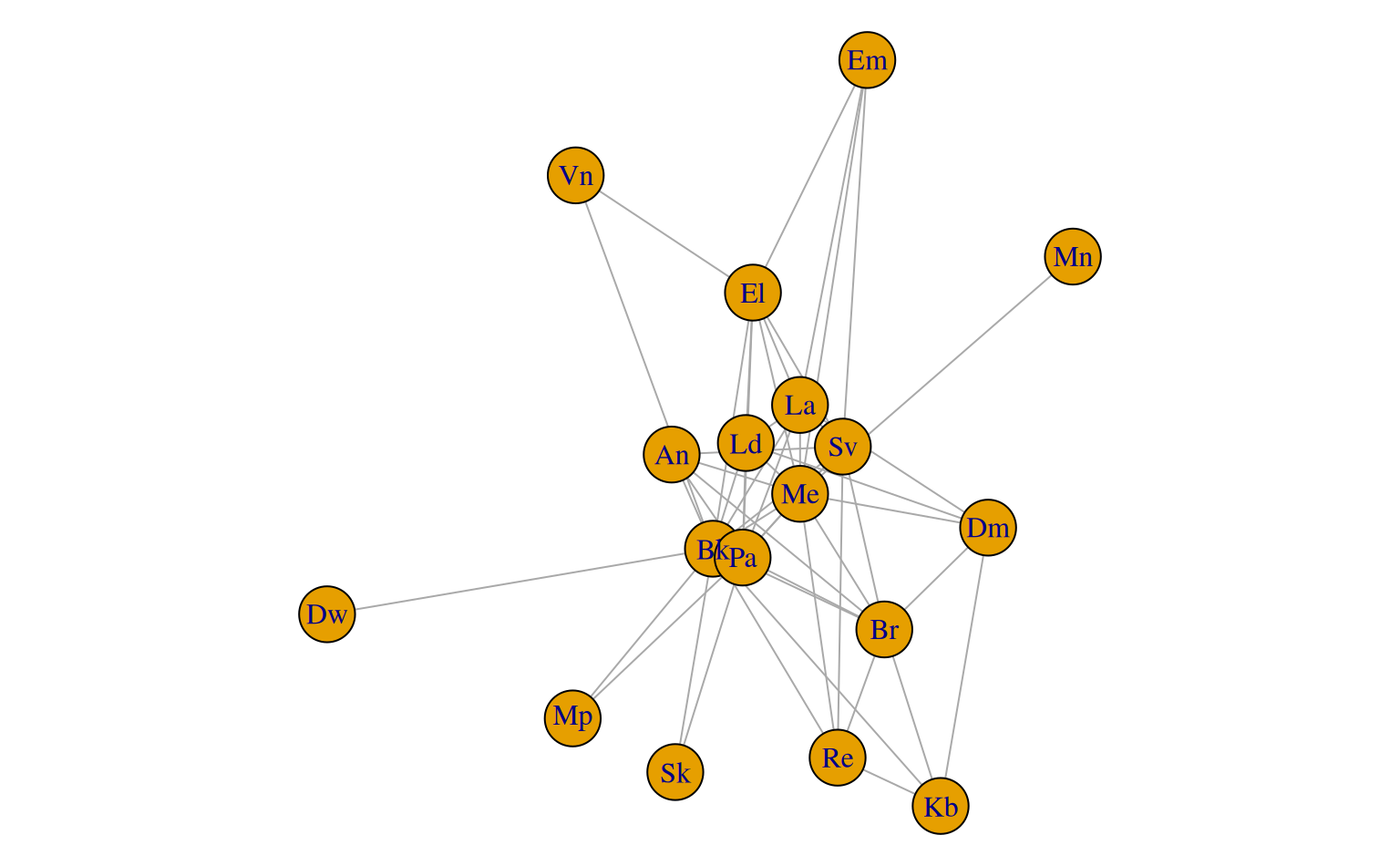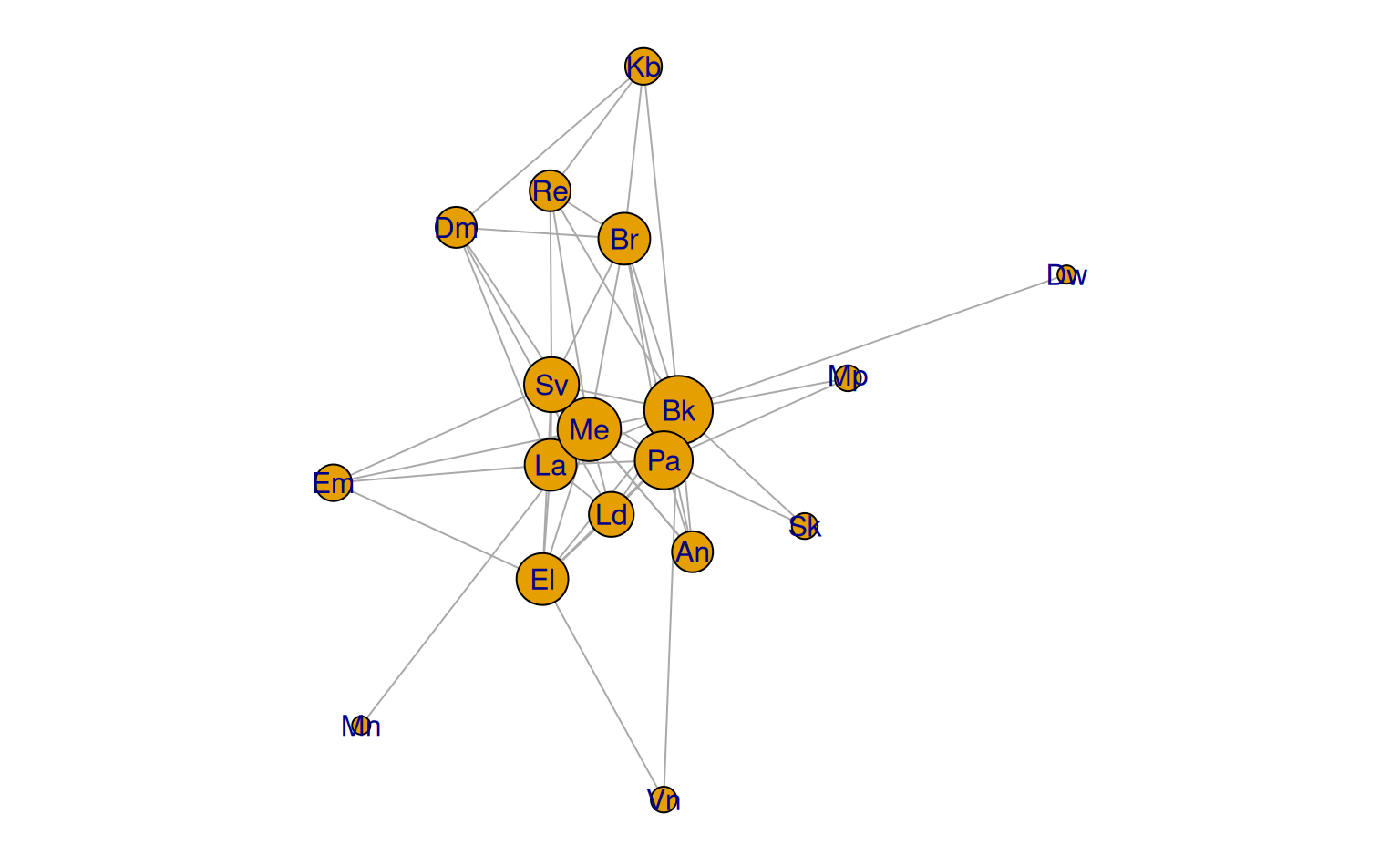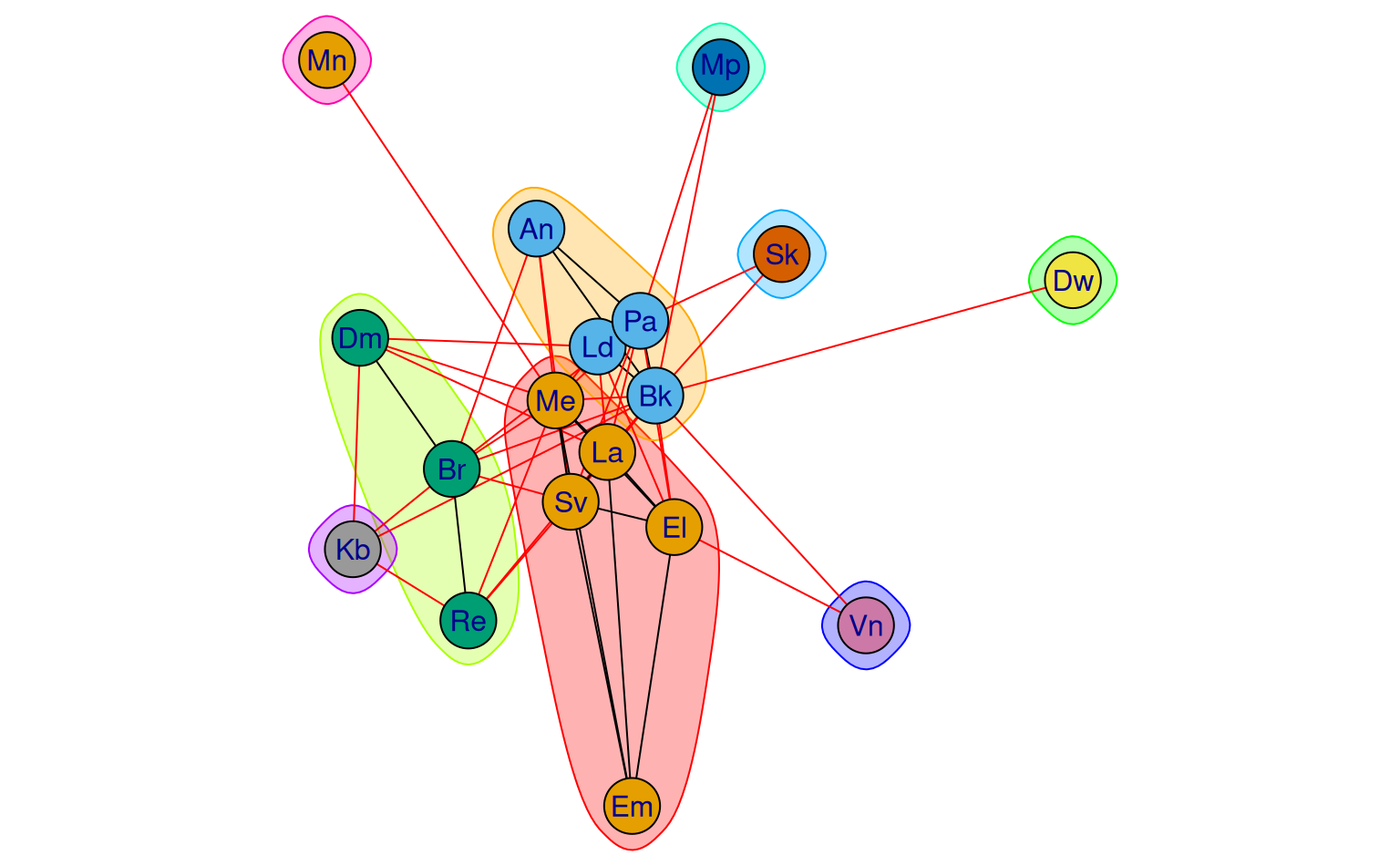An adjacency matrix describing shared soil map boundary segments from the Soil Survey of Shawnee county, KS. This is table 5.2 from Hole and Campbell, 1985.

data(table5.2)

## Format

An object of class matrix (inherits from array) with 18 rows and 18 columns.

## Examples


data("table5.2")

if(requireNamespace("igraph")) {

# note special incantation to get the "correct" graph structure
g <- igraph::graph_from_adjacency_matrix(table5.2, mode = 'upper', diag = FALSE, weighted = TRUE)

# visualize

par(mar = c(0,0,0,0))
plot(g)

plot(g, vertex.size = sqrt(igraph::degree(g) * 25), vertex.label.family = 'sans')

# find communities
cm <- igraph::cluster_walktrap(g)
plot(cm, g, vertex.label.family = 'sans')

par(op)
}